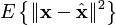# 准备学习Emacs

A Tutorial Introduction to GNU Emacs
http://www2.lib.uchicago.edu/~keith//tcl-course/emacs-tutorial.html
A Emacs+Lisp Tutorial
http://xahlee.org/emacs/emacs.html

# Single Camera Calibration

calibration直接调用opencv里的函数，实现的是zhang’s A flexible new technique for camera calibration的算法，矫正的参数用pov-ray生成的image验证了一把，还是很准的。

location <5, -8, -16>
look_at 0
angle 60
resolution 800×600
right 1.33
up 1

[fx 0 cx]     [692.8203 0 400]
[0 fy cy] =  [0 692.8203 300] (fx = fy = 600 * (2 / 3) * 3 ^ 0.5 = 692.8203.)
[0  0   1]     [0 0        1        ]

calibration的结果为：

693.979 0 399.431
0 692.647 300.201
0 0 1

extrinsic parameters应该为：calibration的结果为：

-0.954613 1.60057e-005 -0.297849
-0.12835 -0.902411 0.411317
-0.268776 0.430877 0.861455

T’ = 0.0100281 -0.000391165 18.578

# Win32程序函数调用时堆栈变化情况分析

1．_stdcall
以__stdcall方式调用的函数有以下特征：
•  参数由右至左压栈
• 调用返回时，堆栈由被调函数调整
2．__cdecl
__cdecl约定是C/C++函数的默认调用约定。它有以下特征：
• 参数由右至左压栈
• 调用返回时，堆栈由调用者调整

1.    压入参数

2.    压入断点

3.    跳转
eip的值被重新设置为被调函数的起始地址。
4.    mov ebp, esp

push ebp

5.    sub esp，N

6.    初始化esp ~ esp-N之间的N字节空间

7.    顺序执行函数内语句。

8．返回

mov esp, ebp

……
0040B8E8   push        1            ;压入参数
0040B8EA   call        00401028        ;调用函数
……
00401028   jmp         0040b7c0        ;跳转到函数入口
……
0040B7C0   push        ebp            ;保存ebp
0040B7C1   mov         ebp,esp
0040B7C3   sub         esp,44h        ;设置函数的堆栈指针，此函数中有4
;字节的局部变量
0040B7C6   push        ebx
0040B7C7   push        esi
0040B7C8   push        edi
0040B7C9   lea         edi,[ebp-44h]
0040B7CC   mov         ecx,11h
0040B7D1   mov         eax,0CCCCCCCCh
0040B7D6   rep stos    dword ptr [edi]    ;初始化局部变量空间
0040B7D8   mov         eax,dword ptr [ebp+8]
0040B7DB   mov         dword ptr [ebp-4],eax
……
0040B7DE   pop         edi            ;弹出曾压栈的数据
0040B7DF   pop         esi
0040B7E0   pop         ebx
0040B7E1   mov         esp,ebp        ;恢复调用者的堆栈
0040B7E3   pop         ebp            ;弹出原ebp值
0040B7E4   ret         4            ;返回并将堆栈向上调整4字节。
;此处为__stdcall约定，所以由函数调
;整堆栈

void __stdcall fun(int);
int main(void)
{
……
fun(1);
……
return 0;
}
void __stdcall fun(int para)
{
int localpara = para;
……
}

# Tail Recursions

http://www.cs.sfu.ca/CC/310/pwfong/Lisp/2/tutorial2.html

Recursive functions are usually easier to reason about. Notice how we articulate the correctness of recursive functions in this and the previous tutorial. However, some naive programmers complain that recursive functions are slow when compared to their iterative counter parts. For example, consider the original implementation of factorial we saw in the previous tutorial:

(defun factorial (N)
"Compute the factorial of N."
(if (= N 1)
1
(* N (factorial (- N 1)))))


It is fair to point out that, as recursion unfolds, stack frames will have to be set up, function arguments will have to be pushed into the stack, so on and so forth, resulting in unnecessary runtime overhead not experienced by the iterative counterpart of the above factorial function:

int factorial(int N) {
int A = 1;
while (N != 1) {
A = A * N;
N = N - 1;
}
return A;
}


Because of this and other excuses, programmers conclude that they could write off recursive implementations …

Modern compilers for functional programming languages usually implement tail-recursive call optimizations which automatically translate a certain kind of linear recursion into efficient iterations. A linear recursive function is tail-recursive if the result of each recursive call is returned right away as the value of the function. Let’s examine the implementation of fast-factorial again:

(defun fast-factorial (N)
"A tail-recursive version of factorial."
(fast-factorial-aux N 1))

(defun fast-factorial-aux (N A)
"Multiply A by the factorial of N."
(if (= N 1)
A
(fast-factorial-aux (- N 1) (* N A))))


Notice that, in fast-factorial-aux, there is no work left to be done after the recursive call (fast-factorial-aux (- N 1) (* N A)). Consequently, the compiler will not create new stack frame or push arguments, but instead simply bind (- N 1) to N and (* N A) to A, and jump to the beginning of the function. Such optimization effectively renders fast-factorial as efficient as its iterative counterpart. Notice also the striking structural similarity between the two.

When you implement linearly recursive functions, you are encouraged to restructure it as a tail recursion after you have fully debugged your implementation. Doing so allows the compiler to optimize away stack management code. However, you should do so only after you get the prototype function correctly implemented. Notice that the technique of accumulator variables can be used even when we are not transforming code to tail recursions. For some problems, the use of accumulator variables offers the most natural solutions.

# OpenCV Matrix指针的玄妙

CvMat* uv = cvCreateMat(16, 2, CV_32FC1);
float* temp = (float*)(uv->data.ptr + 8);

float* temp1 = (float*)(uv->data.ptr + 8 + 4 * 2);

float* temp2 = temp + 8;

# Linear least squares

#### http://en.wikipedia.org/wiki/Linear_least_squares

Limitations
The least squares approach relies on the calculation of the pseudo inverse. The pseudo inverse is guaranteed to exist for any full-rank matrix A. However, in some cases the matrix ATA is ill-conditioned; this occurs when the measurements are only marginally related to the estimated parameters. In these cases, the least squares estimate amplifies the measurement noise, and may be grossly inaccurate. This may occur even when the pseudo inverse itself can be accurately calculated numerically. Various regularization techniques can be applied in such cases, the most common of which is called Tikhonov regularization. If further information about the parameters is known, for example, a range of possible values of x, then minimax techniques can also be used to increase the stability of the solution.

Another drawback of the least squares estimator is the fact that it seeks to minimize the norm of the measurement error, Axb. In many cases, one is truly interested in obtaining small error in the parameter x, e.g., a small value of. However, since x is unknown, this quantity cannot be directly minimized. If a prior probability on x is known, then a Bayes estimator can be used to minimize the mean squared error,. The least squares method is often applied when no prior is known. Surprisingly, however, better estimators can be constructed, an effect known as Stein’s phenomenon. For example, if the measurement error is Gaussian, several estimators are known which dominate, or outperform, the least squares technique; the most common of these is the James-Stein estimator.